The OpenFOAM Foundation
curvedEdge Class Referenceabstract

Define a curved edge that is parameterized for 0<lambda<1 between the start and end point. More...

Inheritance diagram for curvedEdge: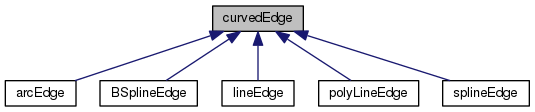[legend]
Collaboration diagram for curvedEdge: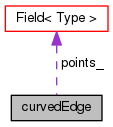[legend]

## Public Member Functions

TypeName ("curvedEdge")
Runtime type information. More...

declareRunTimeSelectionTable (autoPtr, curvedEdge, Istream,(const pointField &points, Istream &is),(points, is))

curvedEdge (const pointField &points, const label start, const label end)
Construct from components. More...

curvedEdge (const pointField &, Istream &)
Construct from Istream setting pointsList. More...

curvedEdge (const curvedEdge &)
Copy construct. More...

virtual autoPtr< curvedEdgeclone () const
Clone function. More...

virtual ~curvedEdge ()
Destructor. More...

label start () const
Return label of start point. More...

label end () const
Return label of end point. More...

int compare (const curvedEdge &) const
Compare the given start and end points with this curve. More...

int compare (const edge &) const
Compare the given start and end points with this curve. More...

int compare (const label start, const label end) const
Compare the given start and end points with this curve. More...

virtual point position (const scalar) const =0
Return the point position corresponding to the curve parameter. More...

virtual scalar length () const =0
Return the length of the curve. More...

void operator= (const curvedEdge &)

## Static Public Member Functions

static autoPtr< curvedEdgeNew (const pointField &, Istream &)
New function which constructs and returns pointer to a curvedEdge. More...

## Static Protected Member Functions

static pointField appendEndPoints (const pointField &, const label start, const label end, const pointField &otherKnots)
Return a complete point field by appending the start/end points. More...

## Protected Attributes

const pointFieldpoints_

const label start_

const label end_

## Friends

Ostreamoperator<< (Ostream &, const curvedEdge &)

## Detailed Description

Define a curved edge that is parameterized for 0<lambda<1 between the start and end point.

Source files

Definition at line 60 of file curvedEdge.H.

## Constructor & Destructor Documentation

 curvedEdge ( const pointField & points, const label start, const label end )

Construct from components.

Definition at line 41 of file curvedEdge.C.

 curvedEdge ( const pointField & points, Istream & is )

Construct from Istream setting pointsList.

Definition at line 53 of file curvedEdge.C.

 curvedEdge ( const curvedEdge & c )

Copy construct.

Definition at line 61 of file curvedEdge.C.

 virtual ~curvedEdge ( )
inlinevirtual

Destructor.

Definition at line 127 of file curvedEdge.H.

Here is the call graph for this function: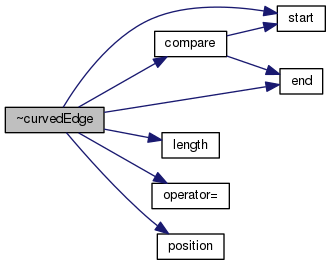## Member Function Documentation

 Foam::pointField appendEndPoints ( const pointField & points, const label start, const label end, const pointField & otherKnots )
staticprotected

Return a complete point field by appending the start/end points.

to the given list

Definition at line 109 of file curvedEdge.C.

References curvedEdge::end(), forAll, List< T >::size(), and curvedEdge::start().

Referenced by curvedEdge::New().

Here is the call graph for this function: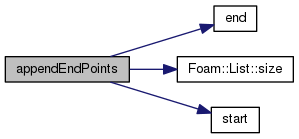Here is the caller graph for this function: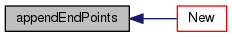TypeName ( "curvedEdge" )

Runtime type information.

 declareRunTimeSelectionTable ( autoPtr , curvedEdge , Istream , (const pointField &points, Istream &is) , (points, is) )
 Foam::autoPtr< Foam::curvedEdge > clone ( ) const
virtual

Clone function.

Definition at line 69 of file curvedEdge.C.

References curvedEdge::New(), and NotImplemented.

Here is the call graph for this function: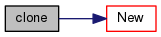Foam::autoPtr< Foam::curvedEdge > New ( const pointField & points, Istream & is )
static

New function which constructs and returns pointer to a curvedEdge.

Definition at line 77 of file curvedEdge.C.

Referenced by curvedEdge::clone().

Here is the call graph for this function: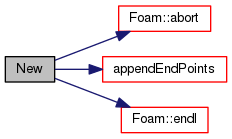Here is the caller graph for this function: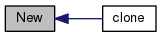Foam::label start ( ) const
inline

Return label of start point.

Definition at line 28 of file curvedEdgeI.H.

References curvedEdge::start_.

Referenced by curvedEdge::appendEndPoints(), curvedEdge::compare(), and curvedEdge::~curvedEdge().

Here is the caller graph for this function: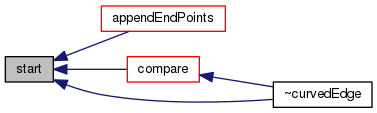Foam::label end ( ) const
inline

Return label of end point.

Definition at line 34 of file curvedEdgeI.H.

References curvedEdge::end_.

Referenced by curvedEdge::appendEndPoints(), curvedEdge::compare(), and curvedEdge::~curvedEdge().

Here is the caller graph for this function: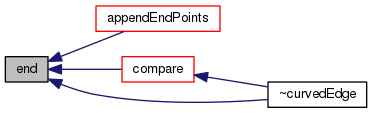int compare ( const curvedEdge & e ) const
inline

Compare the given start and end points with this curve.

Return:

• 0: different
• +1: identical
• -1: same edge, but different orientation

Definition at line 57 of file curvedEdgeI.H.

References curvedEdge::end(), and curvedEdge::start().

Referenced by curvedEdge::compare(), and curvedEdge::~curvedEdge().

Here is the call graph for this function: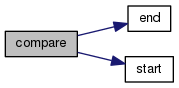Here is the caller graph for this function: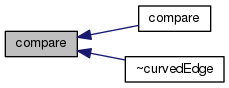int compare ( const edge & e ) const
inline

Compare the given start and end points with this curve.

Return:

• 0: different
• +1: identical
• -1: same edge, but different orientation

Definition at line 63 of file curvedEdgeI.H.

References curvedEdge::compare(), edge::end(), and edge::start().

Here is the call graph for this function: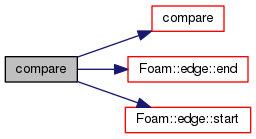int compare ( const label start, const label end ) const
inline

Compare the given start and end points with this curve.

Return:

• 0: different
• +1: identical
• -1: same edge, but different orientation

Definition at line 40 of file curvedEdgeI.H.

References curvedEdge::end_, and curvedEdge::start_.

 virtual point position ( const scalar ) const
pure virtual

Return the point position corresponding to the curve parameter.

0 <= lambda <= 1

Implemented in arcEdge, BSplineEdge, polyLineEdge, splineEdge, and lineEdge.

Referenced by lineDivide::lineDivide(), and curvedEdge::~curvedEdge().

Here is the caller graph for this function: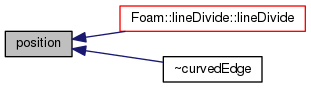virtual scalar length ( ) const
pure virtual

Return the length of the curve.

Implemented in arcEdge, BSplineEdge, polyLineEdge, splineEdge, and lineEdge.

Referenced by curvedEdge::~curvedEdge().

Here is the caller graph for this function: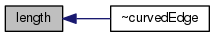void operator= ( const curvedEdge & )

Definition at line 134 of file curvedEdge.C.

References NotImplemented.

Referenced by curvedEdge::~curvedEdge().

Here is the caller graph for this function: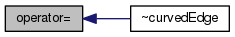## Friends And Related Function Documentation

 Ostream& operator<< ( Ostream & , const curvedEdge & )
friend

Referenced by curvedEdge::~curvedEdge().

## Member Data Documentation

 const pointField& points_
protected

Definition at line 66 of file curvedEdge.H.

Referenced by lineEdge::length(), and lineEdge::position().

 const label start_
protected

Definition at line 67 of file curvedEdge.H.

 const label end_
protected

Definition at line 68 of file curvedEdge.H.

The documentation for this class was generated from the following files: